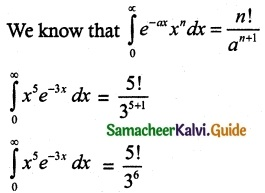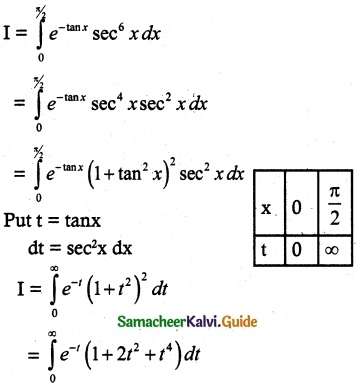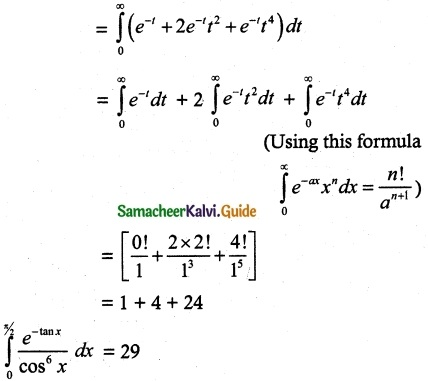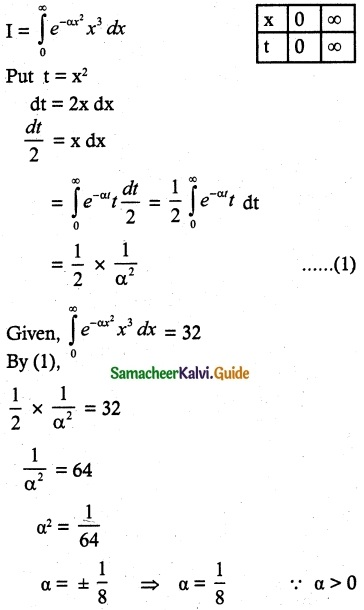Tamilnadu State Board New Syllabus Samacheer Kalvi 12th Maths Guide Pdf Chapter 9 Applications of Integration Ex 9.7 Textbook Questions and Answers, Notes.

## Tamilnadu Samacheer Kalvi 12th Maths Solutions Chapter 9 Applications of Integration Ex 9.7

Question 1.
Evaluate the following
(i) $$\int_{0}^{∞}$$ x5 e-3x dx
Solution:(ii) $$\int_{0}^{π/2}$$ $$\frac{e^{-tanx}}{cos^6 x}$$ dx
Solution:Question 2.
$$\int_{0}^{∞}$$ sinαx² x³ dx = 32, α > 0, find α
Solution: